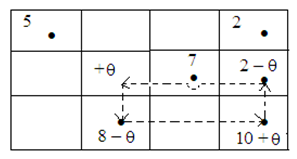#### MODI Method - Transportation Algorithm for Minimization Problem

Transportation Algorithm for Minimization Problem (MODI Method)

Step 1

Make the transportation table entering the origin capacities ai, the cost cij and destination requirement bj

Step 2

Determine an initial basic feasible solution by vogel's technique or by any of the known method.

Step 3

For all the basic variables xij, solve the system of equations ui + vj = cij, for all i, j for which cell (i, j) is in the basis, starting at first with some ui = 0, compute the values of ui and vj on the transportation table

Step 4

Calculate the cost differences dij = cij - ( ui + vj ) for all the non-basic cells

Step 5

Apply optimality test by testing the sign of each dij

• If all dij ≥ 0, the present basic feasible solution is optimal or best
• If at least one dij < 0, choose the variable xrs (most negative) to enter the basis.
• Solution under test is not optimal if any of the dij is negative and subsequent improvement is needed by repeating the above procedure.

Step 6

Assume the variable xrs enter the basis. Assign an unknown quantity ? to the cell (r, s). Then make a loop that begins and ends at the cell (r, s) and links some of the basic cells. The amount ? is added to and subtracted from the transition cells of the loop in such a way that the availabilities and necessities remain fulfilled.

Step 7

Allocate the largest possible value to the ? in such a manner that the value of at least one basic variable comes out to be zero and the other basic variables remain non-negative. The basic cell whose allotment has been made zero will leave the basis.

Step 8

Now, go back to step 3 and repeat the process until an optimal solution is achieved.

Worked Examples

Illustration 1

Determine an optimal solution

 W1 W2 W3 W4 Availability F1 19 30 50 10 7 F2 70 30 40 60 9 F3 40 8 70 20 18 Requirement 5 8 7 14

1. Put vogel's approximation method for determining the initial basic feasible solution

 W1 W2 W3 W4 Availability Penalty F1 5(19) (30) (50) 2(10) X X F2 (70) (30) 7(40) 2(60) X X F3 (40) 8(8) (70) 10(20) X X Requirement X X X X Penalty X X X X

Minimum transportation cost comes out to be 5 (19) + 2 (10) + 7 (40) + 2 (60) + 8 (8) + 10 (20) = Rs. 779

2. Test for Non-degeneracy

The initial fundamental feasible solution has m + n - 1 i.e. 3 + 4 - 1 = 6 allocations in independent positions. Therefore optimality test is fulfilled.

3. Computation of ui and vj : -  ui + vj  = cij

 ui (19) (10) u1= -10 (40) (60) u2 = 40 (8) (20) u3 = 0 vj v1 = 29 v2 = 8 v3 = 0 v4 = 20

Allocate a 'u' value to zero. (Convenient rule is to choose the ui, which has the maximum number of allocations in its row)

Assume u3 = 0, then

u3 + v4= 20 which means 0 + v4 = 20, so v4 = 20

u2 + v4= 60 which means u2 + 20 = 60, so u2 = 40

u1 + v4= 10 which means u1 + 20 = 10, so u1 = -10

u2 + v3= 40 which means 40 + v3 = 40, so v3 = 0

u3 + v2= 8 which means 0 + v2 = 8, so v2 = 8

u1 + v1= 19 which means -10 + v1= 19, so v1 = 29

4. Computation of cost differences for non basic cells dij = cij - ( ui + vj )

 cij ui + vj (30) (50) -2 -10 (70) (30) 69 48 (40) (70) 29 0

 dij = cij - ( ui + vj ) 32 60 1 -18 11 70

5. Doing Optimality test

dij < 0 i.e. d22 = -18

so x22 is entering the basis

6. Creation of loop and allotment of unknown quantity ?We assign ? to the cell (2, 2). Reallocation is done by transferring the maximum possible amount ? in the marked cell. The value of ? is achieved by equating to zero to the corners of the closed loop. That is min (8-?, 2-?) = 0 which gives ? = 2. Hence x24 is outgoing as it turns out to be zero.

 5 (19) 2 (10) 2 (30) 7 (40) 6 (8) 12 (20)

Minimum transportation cost comes out to be 5 (19) + 2 (10) + 2 (30) + 7 (40) + 6 (8) + 12 (20) = Rs. 743

7. Enhanced Solution

 ui (19) (10) u1= -10 (30) (40) u2 = 22 (8) (20) u3 = 0 vj v1 = 29 v2 = 8 v3 = 18 v4 = 20

 cij ui + vj (30) (50) -2 8 (70) (60) 51 42 (40) (70) 29 18

 dij = cij - ( ui + vj ) 32 42 19 18 11 52

As dij > 0, an optimal solution is achieved with minimal cost of Rs.743